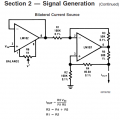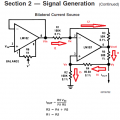# Bilateral Current Source Analysis

#### BramLabs

Joined Nov 21, 2013
98
Here's the picture of the circuit :And here's my analysis so far :First equation i get :
i1 = i2
(Vin - Vx)/R1 = (Vx - Vout)/R3

Second equation i get :
i4 = i5
(Vy - Vx)/R4 = Vx/R2

Third equation i get :
Iout = -i4 + i3

What makes me confuse that i've tried to make Second equation to get Vx = ........
Then, when i applied it to the first equation i got 3 variable, and i think that i made it even worst.

So, i'm now up to these ( second equation ) :
i4 = i5
(Vy - Vx)/R4 = Vx/R2
Vy/R4 = Vx/R2 + Vx/R4
Vy/R4 = Vx( (1/R2) + (1/R4) )
Then i don't know, whether to make Vx = ..... or Vy = ....

#### WBahn

Joined Mar 31, 2012
28,173
See if you can come up with the following relationships:

Vout in terms of Vx and Vin

Vx in terms of Vy

Iout in terms of Vout, Vy and Vx

This would give you three equations in the unknowns (Vin, Vout, Vx, Vy, and Iout) allowing you to come up with one equation that eliminates three of them yielding an equation that relates Iout to Vin.

•BramLabs

#### anhnha

Joined Apr 19, 2012
901
•BramLabs

#### The Electrician

Joined Oct 9, 2007
2,916
Jony, in the link you assumed opamp is ideal and for that reason Vn = Vp.
I have some problems hope you can clarify.
In the circuit both positive and negative feedback configurations are present, so for a normal opamp (very high gain but not infinity) if I understand correctly Vn = Vp only if negative feedback is stronger than the positive one?

•BramLabs and anhnha

#### RBR1317

Joined Nov 13, 2010
706
Cranking out the algebra solution is not very interesting, except for the part where it is necessary to eliminate the Vout variable. The method to do that is to balance out the negative and positive coefficients of Vout so they cancel any effect from Vout in the final equation. I have attached a worksheet from a symbolic algebra engine which illustrates this.

However, I did notice the attempt to perform a nodal analysis using the node currents explicitly. That seems to me an awkward approach since there is a method for writing the node equations in terms of the node voltages that applies Kirchhoff's current law implicitly. That method is used to write the three node equations for the circuit on the attached worksheet.

Each term of the node equation begins with the node voltage for that node. Each path to an adjacent node has one term in the node equation, and the sum of all terms will equal zero. Each term is formed in the following manner: Node voltage minus adjacent node voltage, all divided by the resistance (or impedance) in the path from the node to the adjacent node. With these conventions, current leaving a node is considered to be positive. Each term represents the flow of current between the nodes, but only node voltages are calculated. Of course, current sources must be accounted for directly. I find it difficult to make a mistake when writing node equations using this method.

#### Attachments

•BramLabs

#### The Electrician

Joined Oct 9, 2007
2,916
Cranking out the algebra solution is not very interesting, except for the part where it is necessary to eliminate the Vout variable. The method to do that is to balance out the negative and positive coefficients of Vout so they cancel any effect from Vout in the final equation. I have attached a worksheet from a symbolic algebra engine which illustrates this.

However, I did notice the attempt to perform a nodal analysis using the node currents explicitly. That seems to me an awkward approach since there is a method for writing the node equations in terms of the node voltages that applies Kirchhoff's current law implicitly. That method is used to write the three node equations for the circuit on the attached worksheet.

Each term of the node equation begins with the node voltage for that node. Each path to an adjacent node has one term in the node equation, and the sum of all terms will equal zero. Each term is formed in the following manner: Node voltage minus adjacent node voltage, all divided by the resistance (or impedance) in the path from the node to the adjacent node. With these conventions, current leaving a node is considered to be positive. Each term represents the flow of current between the nodes, but only node voltages are calculated. Of course, current sources must be accounted for directly. I find it difficult to make a mistake when writing node equations using this method.
The philosophy of the homework help forum is to not completely solve the problem for the TS, but to guide them to their own solution.

#### RBR1317

Joined Nov 13, 2010
706
...guide them to their own solution.
The TS already had the solution. What the TS did not have is a foolproof method for writing a system of node equations. Nor did the TS have a symbolic algebra engine to crank out the algebra for a system of node equations.

#### The Electrician

Joined Oct 9, 2007
2,916
The TS already had the solution. What the TS did not have is a foolproof method for writing a system of node equations. Nor did the TS have a symbolic algebra engine to crank out the algebra for a system of node equations.
The TS had the final answer. The "solution" I was referring to was the formulation of a suitable set of equations and then solving them. That's what you did for him, rather than guiding him to that goal.

#### BramLabs

Joined Nov 21, 2013
98
Thanks for your help sir !

See if you can come up with the following relationships:

Vout in terms of Vx and Vin

Vx in terms of Vy

Iout in terms of Vout, Vy and Vx

This would give you three equations in the unknowns (Vin, Vout, Vx, Vy, and Iout) allowing you to come up with one equation that eliminates three of them yielding an equation that relates Iout to Vin.
Okay sir, i'll try !
Thanks btw for your help !

Thanks for your help sir !

Cranking out the algebra solution is not very interesting, except for the part where it is necessary to eliminate the Vout variable. The method to do that is to balance out the negative and positive coefficients of Vout so they cancel any effect from Vout in the final equation. I have attached a worksheet from a symbolic algebra engine which illustrates this.

However, I did notice the attempt to perform a nodal analysis using the node currents explicitly. That seems to me an awkward approach since there is a method for writing the node equations in terms of the node voltages that applies Kirchhoff's current law implicitly. That method is used to write the three node equations for the circuit on the attached worksheet.

Each term of the node equation begins with the node voltage for that node. Each path to an adjacent node has one term in the node equation, and the sum of all terms will equal zero. Each term is formed in the following manner: Node voltage minus adjacent node voltage, all divided by the resistance (or impedance) in the path from the node to the adjacent node. With these conventions, current leaving a node is considered to be positive. Each term represents the flow of current between the nodes, but only node voltages are calculated. Of course, current sources must be accounted for directly. I find it difficult to make a mistake when writing node equations using this method.
I think my method for node equation are the same as you refer to, but in 'different' way.
Because for the first equation, i got the same Vx equation as you have mentioned above.
And for the next step, i'm still working on it. ahahaha... got no clue how to minimize the equation for making Vy = .........

By the way, thank you very much for your answer sir. It does really help me to open my mind. I'm not cheating by looking to your answer, i'm just learning the way you were thinking fro the answer. Find Vx = ..... then Vy = ..... After that, find Iout = ....... Now i understand 'the way' to solve the problem and the critical thinking of why should we find Vx first not Vy.
Thank you very much, i'll upload my answer if i already finish to minimize the equationahahaha...

God bless you all !

#### RBR1317

Joined Nov 13, 2010
706
Because for the first equation, i got the same Vx equation as you have mentioned above.
There would be something terribly wrong if we got different node equations for the same circuit! The advantage of following 'the method' is that one can write the node equations without thinking much about it. In my experience it is thinking too much that leads to mistakes.

Now i understand 'the way' to solve the problem and the critical thinking of why should we find Vx first not Vy.
On further reflection it seems that it would be simpler to find Vy first and eliminate it by substitution, then eliminate Vx. Either approach will yield the same expression for Iout. With a symbolic algebra engine one can go the more complicated route because the computer does all the work and it doesn't complain.

#### MrAl

Joined Jun 17, 2014
9,773
Jony, in the link you assumed opamp is ideal and for that reason Vn = Vp.
I have some problems hope you can clarify.
In the circuit both positive and negative feedback configurations are present, so for a normal opamp (very high gain but not infinity) if I understand correctly Vn = Vp only if negative feedback is stronger than the positive one?
Hi,

I got into this discussion a little later, but did you mean that you also wanted to include the actual gain of the op amp in the equations?